# Benchmarking in Julia

In Julia, most of the codes are checked for it’s speed and efficiency. One of the hallmarks of Julia is that it’s quite faster than it’s other scientific computing counterparts(Python, R, Matlab). To verify this, we often tend to compare the speed and performance of a code block run across various languages. In cases where we try out multiple methods to solve a problem, and it becomes necessary to decide the most efficient approach, in that case we obviously choose the fastest method.

One of the most conventional ways to test out a code block in Julia is using the @time macro. In Julia we say that global objects tend to decrease the performance.

Also, since we use randomly generated values, we will seed the RNG, so that the values are consistent between trials/samples/evaluations.

 `# Import the library ` `using Random ` `   `  `# Using the MersenneTwister rng ` `# Here 1234 is a seed value ` `rng ``=` `MersenneTwister(``1234``); ` `  `  `# Generate random data ` `x ``=` `rand(rng, ``1000``); ` `  `  `# a function that considers ` `# x as a global variable ` `function prod_global() ` `    ``prod ``=` `0.0` `    ``for` `i ``in` `x ` `       ``prod ``*``=` `i ` `    ``end ` `    ``return` `prod ` `end; ` `  `  `# a function that accepts ` `# x as a local variable ` `function prod_local(x) ` `    ``prod ``=` `0.0` `    ``for` `i ``in` `x ` `       ``prod ``*``=` `i ` `    ``end ` `    ``return` `prod ` `end; `

Output: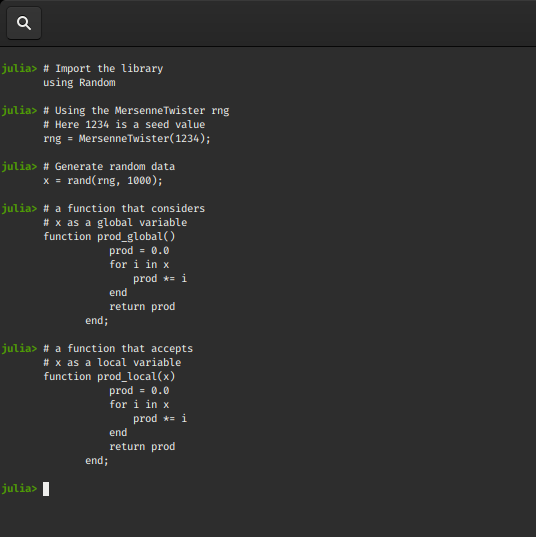Now to compare the two functions we will use our @time macro. For a fresh environment, on the first call (@time prod_global()), the prod_global() function and other functions needed for timing are compiled so the results of first run shouldn’t be taken seriously.

 `# first run ` `@time` `prod_global() ` ` `  `# second run ` `@time` `prod_global() `

Output: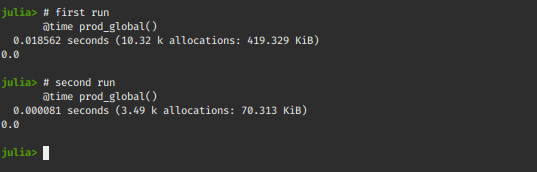Let’s try to test the function with a local x

 `# first run ` `@time` `prod_local(x) ` ` `  `# second run ` `@time` `prod_local(x) `

Output: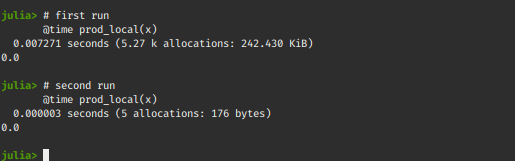#### Profiling Julia Code

For profiling code in Julia we use the @profile macro. It performs measurements on running code, and produces output that helps developers analyze the time spent per line. It is generally used to identify bottlenecks in code blocks/functions that hinder performance.

Let’s try to profile our previous example and see why global variables hinder performance!
Also, we will replace the product by sum now, so that the calculations don’t tend towards infinity or zero at any point.

 `# Import the library ` `using Random ` `   `  `# Using the MersenneTwister rng ` `# Here 1234 is a seed value ` `rng ``=` `MersenneTwister(``1234``); ` `  `  `# Generate random data ` `x ``=` `rand(rng, ``1000``); ` `  `  `# a function that considers ` `# x as a global variable ` `function sum_global() ` `    ``sum` `=` `0.0` `    ``for` `i ``in` `x ` `       ``sum` `+``=` `i ` `    ``end ` `    ``return` `sum` `end; ` ` `  `# First we force compile the function ` `sum_global() ` `  `  `# Import profiling library ` `using Profile ` `  `  `# Profile sum_global ` `@profile` `sum_global ` `  `  `# Print the results ` `Profile.``print``() `

Output: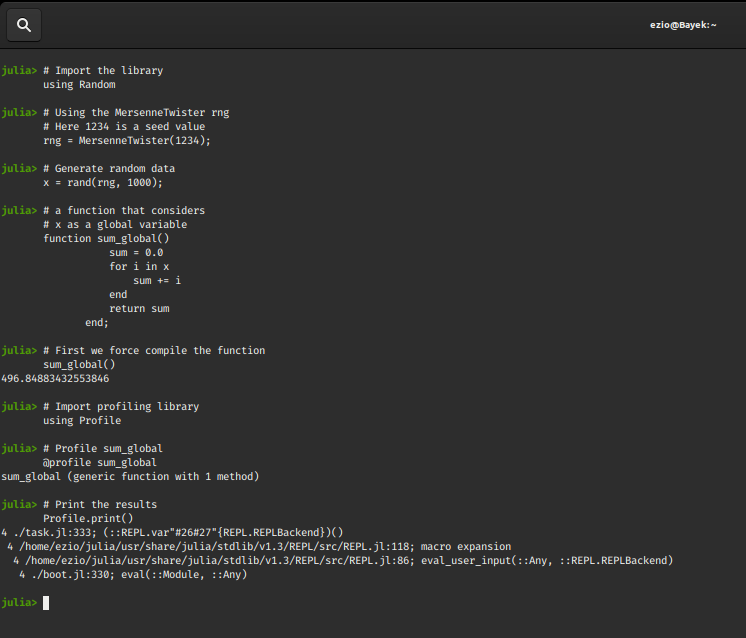`# Make sure you run the following code ` `# in a fresh repl environment ` `# This will clear results from preivous profiling ` `# Import the library ` `using Random ` `   `  `# Using the MersenneTwister rng ` `# Here 1234 is a seed value ` `rng ``=` `MersenneTwister(``1234``); ` `  `  `# Generate random data ` `x ``=` `rand(rng, ``1000``); ` `  `  `# A function that accepts ` `# x as a local variable ` `function sum_local(x) ` `    ``sum` `=` `0.0` `    ``for` `i ``in` `x ` `       ``sum` `+``=` `i ` `    ``end ` `    ``return` `sum` `end; ` `  `  `# Force compile the function ` `sum_local(x) ` `  `  `# Import the library  ` `using Profile ` `  `  `# Profile sum_local() ` `@profile` `sum_local(x) ` `  `  `# Print the results ` `Profile.``print``() `

Output: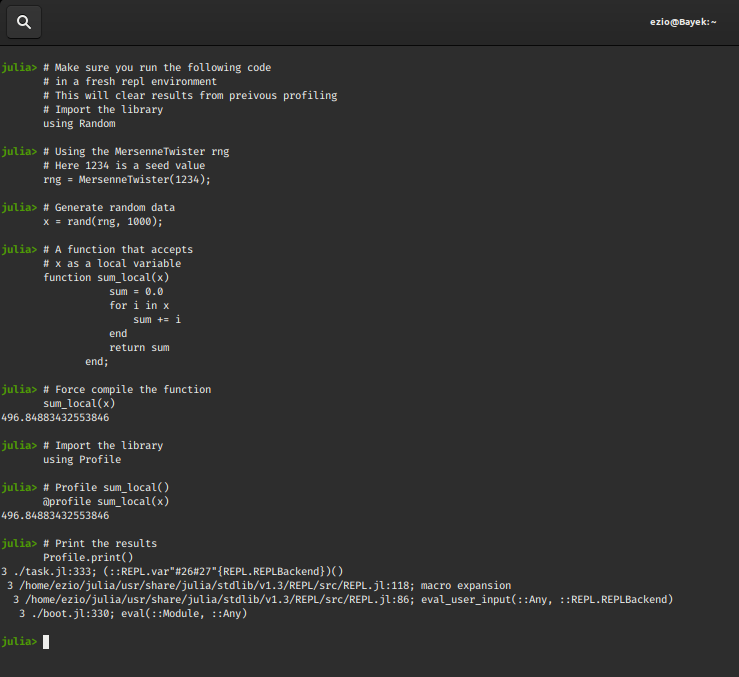You must be wondering that how can we simply conclude the performance of a code based on @time and profiling on one go and many of such decisions are made by consistent analysis across various trials and observing the code block’s performance over time. Julia has an extension package to run reliable benchmarks called Benchmark Tools.jl

#### Benchmarking a Code

One of the most conventional ways to benchmark a code block using Benchmark Tools is the @benchmark macro
Considering the above example of sum_local(x) and sum_global():

 `# Import the library ` `using Random ` `   `  `# Using the MersenneTwister rng ` `# Here 1234 is a seed value ` `rng ``=` `MersenneTwister(``1234``); ` `  `  `# Import the package ` `using BenchmarkTools ` `  `  `# Generate random data ` `x ``=` `rand(rng, ``1000``); ` `  `  `# a function that considers ` `# x as a global variable ` `function sum_global() ` `    ``sum` `=` `0.0` `    ``for` `i ``in` `x ` `       ``sum` `+``=` `i ` `    ``end ` `    ``return` `sum` `end; ` `  `  `# A function that accepts ` `# x as a local variable ` `function sum_local(x) ` `    ``sum` `=` `0.0` `    ``for` `i ``in` `x ` `       ``sum` `+``=` `i ` `    ``end ` `    ``return` `sum` `end; ` ` `  `# Benchmark the sum_global() function ` `@benchmark` `sum_global() ` `  `  `# Benchmark the sum_local(x) function ` `@benchmark` `sum_local(x)  `

Output: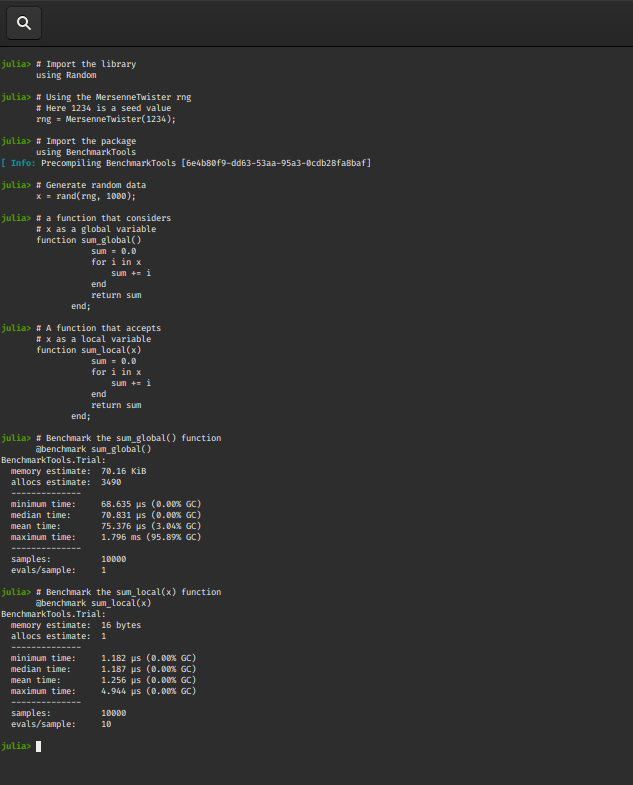The @benchmark macro gives out a lot of details(mem. allocs, minimum time, mean time, median time, samples etc.) that come in handy for many developers, but there are times when we need a quick specific reference, eg : the @btime macro prints the minimum time and memory allocation before returning the value of the expression and the @belapsed macro returns the minimum time in seconds.

 `# @btime for sum_global() ` `@btime` `sum_global()  ` ` `  `# @btime for sum_local(x) ` `@btime` `sum_local(x) ` ` `  `# @belapsed for sum_global() ` `@belapsed` `sum_global() ` ` `  `# @belapsed for sum_local(x) ` `@belapsed` `sum_local(x) `

Ouptut: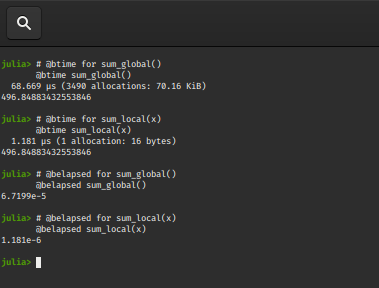The @benchmark macro offers us ways to configure the benchmark process.
You can pass the following keyword arguments to @benchmark, and run to configure the execution process:

• samples: It determines the number of samples to take and defaults to
BenchmarkTools.DEFAULT_PARAMETERS.samples = 10000.
• seconds: The number of seconds allocated for the benchmarking process. The trial will terminate if this time is exceeded irrespective of number of samples, but atleast one sample will always be taken.
It defaults to BenchmarkTools.DEFAULT_PARAMETERS.seconds = 5.
• evals: It determines the number of evaluations per sample. It defaults to
BenchmarkTools.DEFAULT_PARAMETERS.evals = 1.
• overhead: The estimated loop overhead per evaluation in nanoseconds, which is automatically subtracted from every sample time measurement. The default value is
• gctrial: If true, run gc() (garbage collector)before executing the benchmark’s trial.
Defaults to BenchmarkTools.DEFAULT_PARAMETERS.gctrial = true.
• gcsample: If set true, run gc() before each sample.
Defaults to BenchmarkTools.DEFAULT_PARAMETERS.gcsample = false.
• time_tolerance: The noise tolerance for the benchmark’s time estimate, as a percentage. This is utilized after benchmark execution, when analyzing results.
Defaults to BenchmarkTools.DEFAULT_PARAMETERS.time_tolerance = 0.05.
• memory_tolerance: The noise tolerance for the benchmark’s memory estimate, as a percentage. This is utilized after benchmark execution, when analyzing results.
Defaults to BenchmarkTools.DEFAULT_PARAMETERS.memory_tolerance = 0.01.

 `# apply custom benchmarks ` `bg ``=` `@benchmark sum_global() seconds``=``1` `time_tolerance``=``0.01` ` `  `# apply custom benchmarks ` `bl ``=` `@benchmark sum_local(x) seconds``=``1` `time_tolerance``=``0.01`

Output: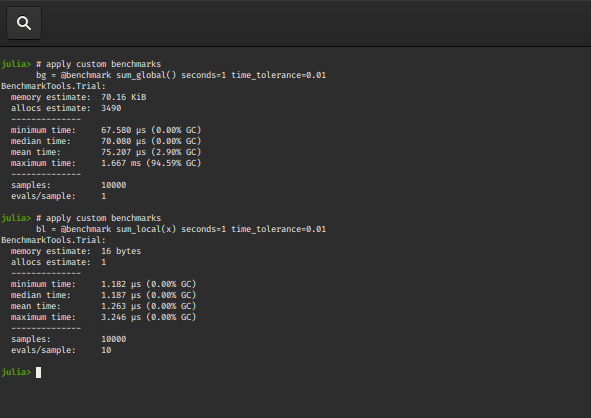My Personal Notes arrow_drop_upCheck out this Author's contributed articles.

If you like GeeksforGeeks and would like to contribute, you can also write an article using contribute.geeksforgeeks.org or mail your article to contribute@geeksforgeeks.org. See your article appearing on the GeeksforGeeks main page and help other Geeks.

Please Improve this article if you find anything incorrect by clicking on the "Improve Article" button below.

Article Tags :

Be the First to upvote.

Please write to us at contribute@geeksforgeeks.org to report any issue with the above content.